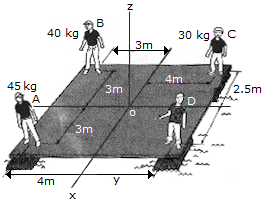# Engineering Mechanics - Force System Resultants - Discussion

### Discussion :: Force System Resultants - General Questions (Q.No.8)

8.The boys A, B and C stand near the edges of a raft as shown. Determine the location (x, y) of boy D so that all four boys create a single resultant force acting through the raft's center O. Provided the raft itself is symmetric, this would keep the raft afloat in a horizontal plane. the mass of each boy is indicated in the diagram.

 [A]. x = 4.5 m, y = 1.5 m [B]. x = 3.0 m, y = 3.0 m [C]. x = 1.5 m, y = 4.5 m [D]. x = 3.0 m, y = 4.0 m

Explanation:

No answer description available for this question.

 Virendra said: (May 9, 2017) I think Option A is the right one.